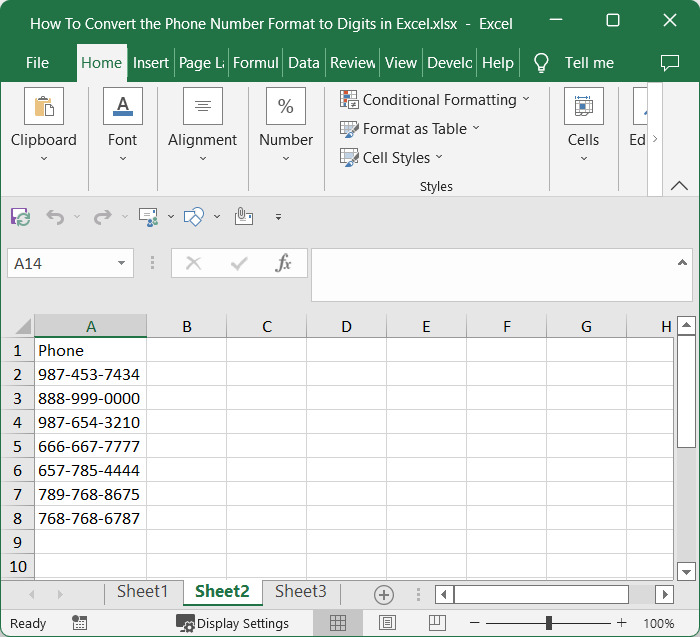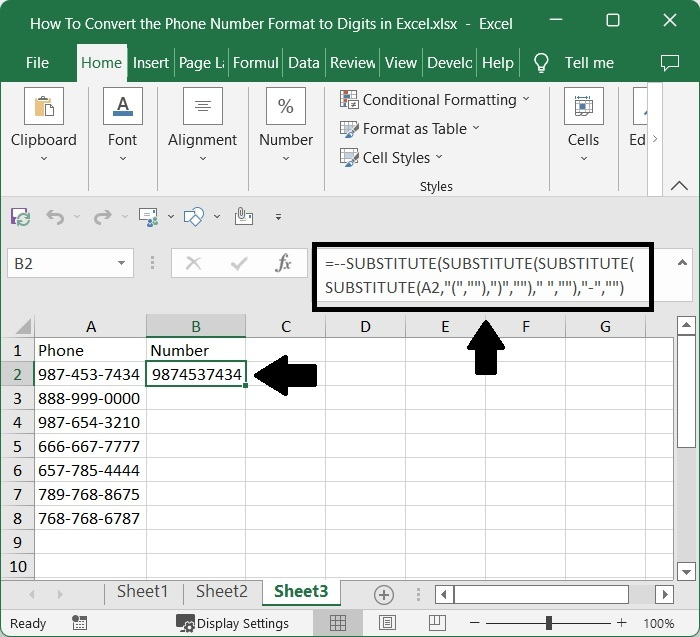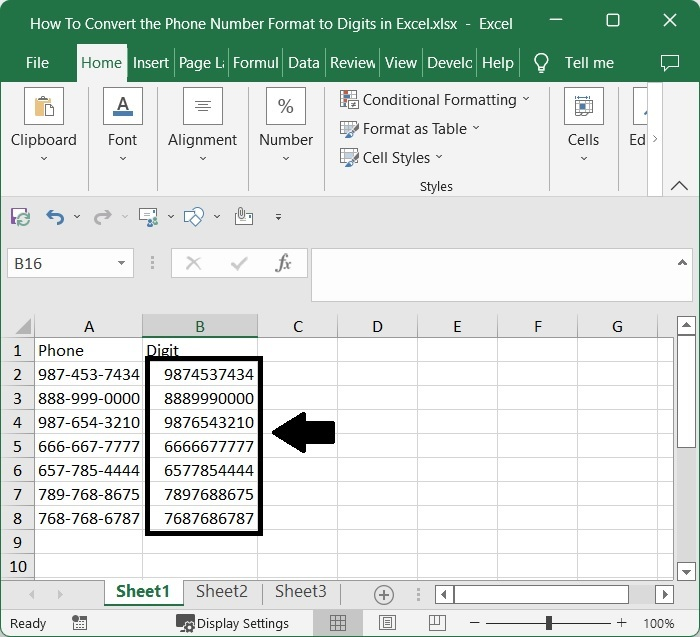# How to Convert the Phone Number Format to Digits in Excel?

Generally, when we need to collect mobile phone numbers on an excel sheet, the values are stored in a special format to differentiate them from normal digits. The special format is a "-" separator used after the third and sixth digits in the ten-digit phone number. However, you may need to convert those phone numbers to standard digit format on occasion. If we try to do that manually, then it can be a complex and time-consuming task. Using the formula, we can complete the task more quickly and easily.

Read this tutorial to learn how you can convert phone number format to digits in Excel. We can use the SUBSTITUTE function to complete our task in a simple way. The Excel SUBSTITUTE function replaces text in a given string by matching. For example, =SUBSTITUTE("952-455-7865","-","") returns "9524557865";

The dash is stripped. SUBSTITUTE is case-sensitive and does not support wildcards.

## Converting the Phone Number Format to Digits in Excel

Here, we will first use the loop of SUBSTITUTE formulas to get any one of the results and then use the auto-fill handle to get all the results.

### Step 1

Consider any excel sheet where the data is a list of phone numbers, as shown in the image below.Then, in our case, cell B2, click on an empty cell and enter the formula as follows −

=--SUBSTITUTE(SUBSTITUTE(SUBSTITUTE(SUBSTITUTE(A2,"(",""),")","")," ",""),"-","")and click enter to get our first result as similar to below image. In the formula, A2, there is the address of the phone number in the Excel sheet.

Empty cell > Formula > Enter### Step 2

Then drag down from the first result using the auto-fill handle, and our final result will be similar to the below image.## Conclusion

In this tutorial, we used a simple example to demonstrate how you can convert a phone number to digits in Excel.